Function Repository Resource:

# IdentityTensorReduce

Simplify expressions containing a symbolic identity tensor

Contributed by: Carl Woll
 ResourceFunction["IdentityTensorReduce"][expr] converts TensorContract expressions containing symbolic identity tensors into equivalent forms with the identity tensors removed.

## Details and Options

ResourceFunction["IdentityTensorReduce"] makes no assumptions about the rank of the symbolic tensors involved. Use the Assumptions option or \$Assumptions to define rank information for the involved tensors.
The input and output of ResourceFunction["IdentityTensorReduce"] will evaluate to the same array when given explicit arrays as input.

## Examples

### Basic Examples (2)

A simple tensor contraction of a tensor with an identity matrix:

 In:=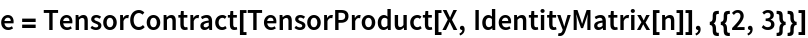Out=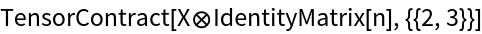TensorReduce does not simplify the tensor:

 In:=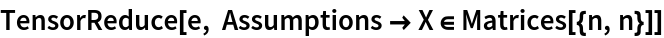Out=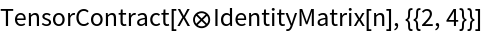IdentityTensorReduce simplifies the tensor:

 In:=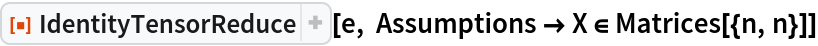Out=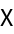Use Inactive when the dimensions of the identity tensor are explicit:

 In:=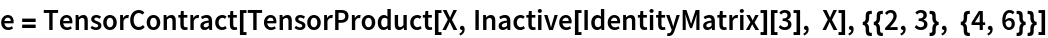Out=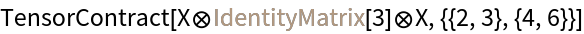IdentityTensorReduce simplifies the tensor:

 In:=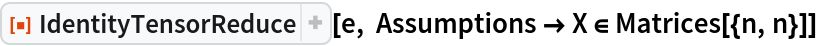Out=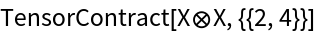### Scope (6)

Define assumptions for a matrix X and scalars a, b and c:

 In:=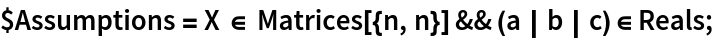A tensor expression involving the identity tensor:

 In:=Out=Contract this tensor with another tensor:

 In:=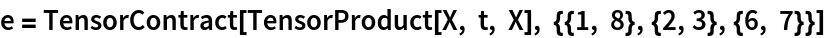Out=Expand the tensor into a sum of tensors:

 In:=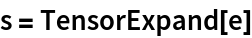Out=Use IdentityTensorReduce to eliminate contracted identity tensors:

 In:=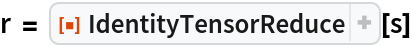Out=The ResourceFunction "FromTensor" can be used to rewrite this expression in terms of Dot:

 In:=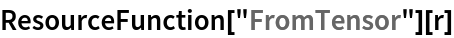Out=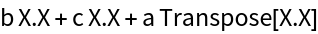Carl Woll

## Version History

• 1.0.0 – 09 November 2020